# Math in Focus Grade 2 Chapter 3 Answer Key Subtraction up to 1,000

Go through the Math in Focus Grade 2 Workbook Answer Key Chapter 3 Subtraction up to 1,000 to finish your assignments.

## Math in Focus Grade 2 Chapter 3 Answer Key Subtraction up to 1,000

challenging Practice

Write the missing numbers.

Question 1.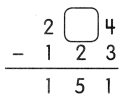The missing number is 7.

Explanation:
In the above image, we can see that the difference and subtrahend. So to find the missing number we will add the difference and the subtrahend. Add 151+123 which is 274, so the missing number is 7.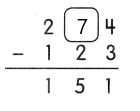Question 2.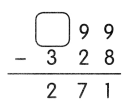The missing number is 5.

Explanation:
In the above image, we can see that the difference and subtrahend. So to find the missing number we will add the difference and the subtrahend. Add 271+328 which is 599, so the missing number is 5.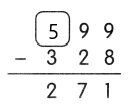Question 3.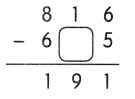The missing number is 2.

Explanation:
In the above image, we can see that the difference and minuend. So to find the missing number we will subtract the minuend and the difference, subtract 816-191 which is 625, so the missing number is 2.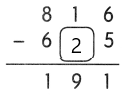Question 4.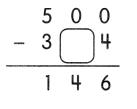The missing number is 5.

Explanation:
In the above image, we can see that the difference and minuend. So to find the missing number we will subtract the minuend and the difference, subtract 500-146 which is 354, so the missing number is 5.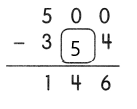Question 5.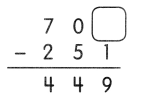The missing number is 0.

Explanation:
In the above image, we can see that the difference and subtrahend. So to find the missing number we will add the difference and the subtrahend. Add 449+251 which is 700, so the missing number is 0.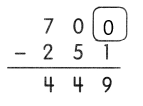Question 6.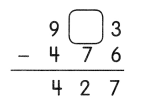The missing number is 0.

Explanation:
In the above image, we can see that the difference and subtrahend. So to find the missing number we will add the difference and the subtrahend. Add 476+427 which is 903, so the missing number is 0.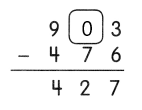Solve.

Question 7.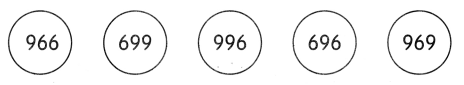a. Write the numbers in order from greatest to least.
996, 969, 966, 699, 696.

Explanation:
The numbers are in order from greatest to least is 996, 969, 966, 699, 696.

b. Subtract the least number from the greatest number. Show your work.
300.

Explanation:
Here, the greatest number is 996 and the least number is 696. So the subtraction between them is 996-696 which is 300.

Fill in the blanks with the numbers below.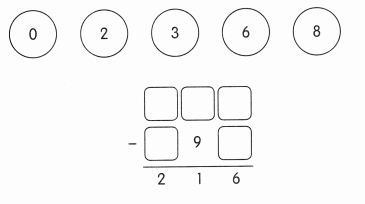606-390 = 216.

Explanation:
Here, 606-390 is 216.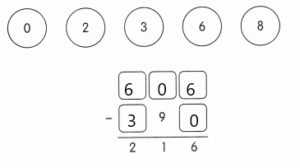Chapter Review/Test

Vocabulary

Question 1.
Fill in the blanks with words from the box. The words may be used more than once.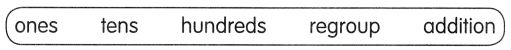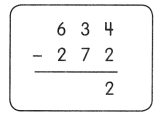Step 1
Subtract the ______.
4 ones – 2 ones = 2 ones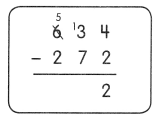As 7 tens cannot be subtracted from 3 tens.
_____________ the hundreds and __________
6 hundreds 3 tens 5 ____________ 13 tens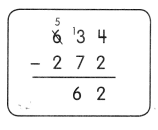Step 2
Subtract the ___.
13 tens – 7 tens = 6 tens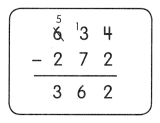Step 3
Subtract the ____.
5 hundreds – 2 hundreds = 3 hundreds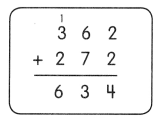If 634 – 272 = 362, then 362 + 272 = 634.
634 – 272 = 362

Explanation:
Step 1
Subtract the ones.
4 ones – 2 ones = 2 onesAs 7 tens cannot be subtracted from 3 tens.
Regroup the hundreds and then subtract.
6 hundreds 3 tens 5 ____________ 13 tensStep 2
Subtract thetens.
13 tens – 7 tens = 6 tensStep 3
Subtract the hundreds.
5 hundreds – 2 hundreds = 3 hundredsIf 634 – 272 = 362, then 362 + 272 = 634.

Concepts and Skills

Subtract
Then match those with the same answer.

Question 2.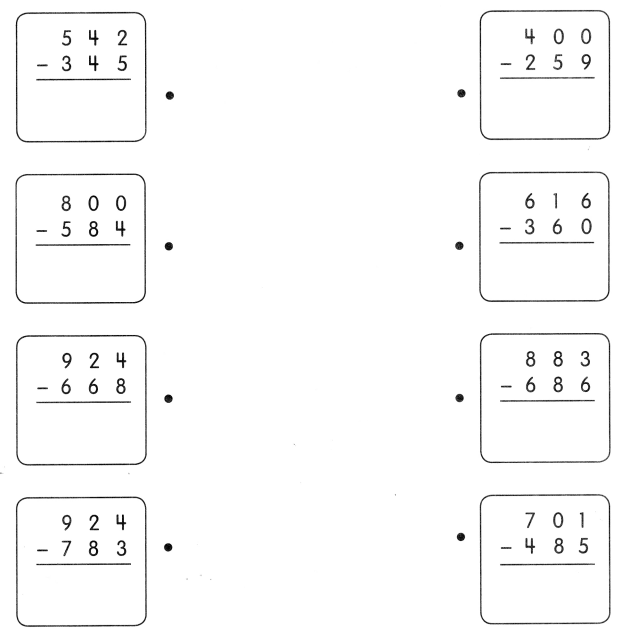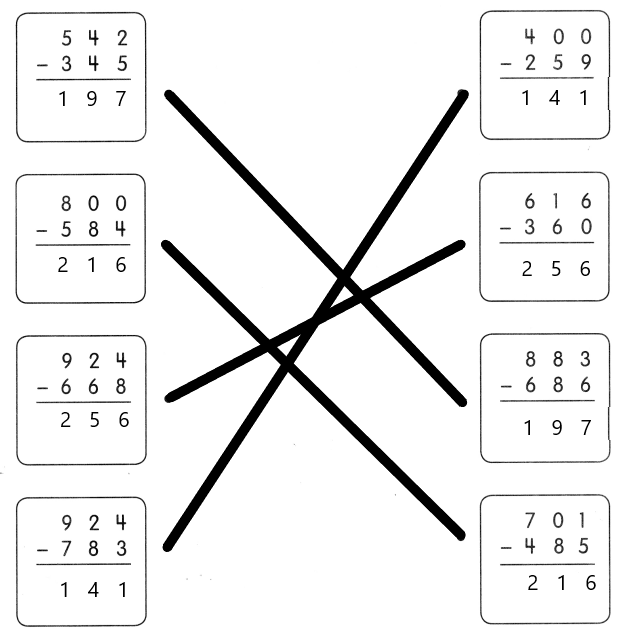Problem Solving

Solve.

Question 3.
A supermarket has 4-12 bottles of apple juice. 123 bottles of apple juice are sold. How many bottles of apple juice are left?
___ bottles of apple juice are left.

Question 4.
Mr. Smith made 207 sandwiches. 18 sandwiches are tuna. How many sandwiches are not tuna?
____ sandwiches are not tuna.
189 sandwiches are not tuna.

Explanation:
Given that Mr. Smith made 207 sandwiches and 18 sandwiches are tuna. So the number of sandwiches are not tuna is 207-18 which is 189 sandwiches.

Question 5.
The Morgans drive 864 miles in the first week of their vacation. They drive 178 fewer miles in the second week. How many miles do they drive in the second week?
They drive ____ miles in the second week.